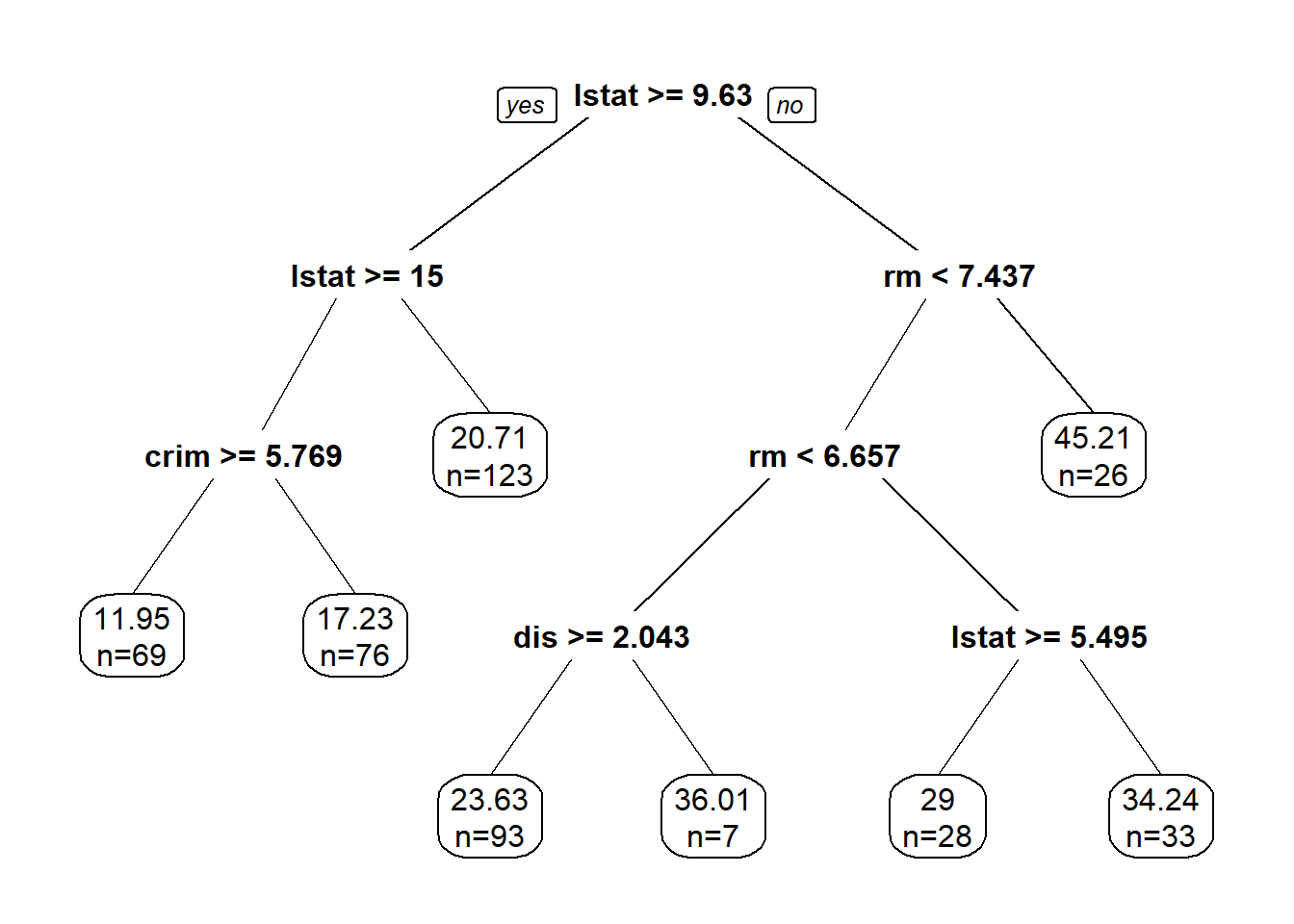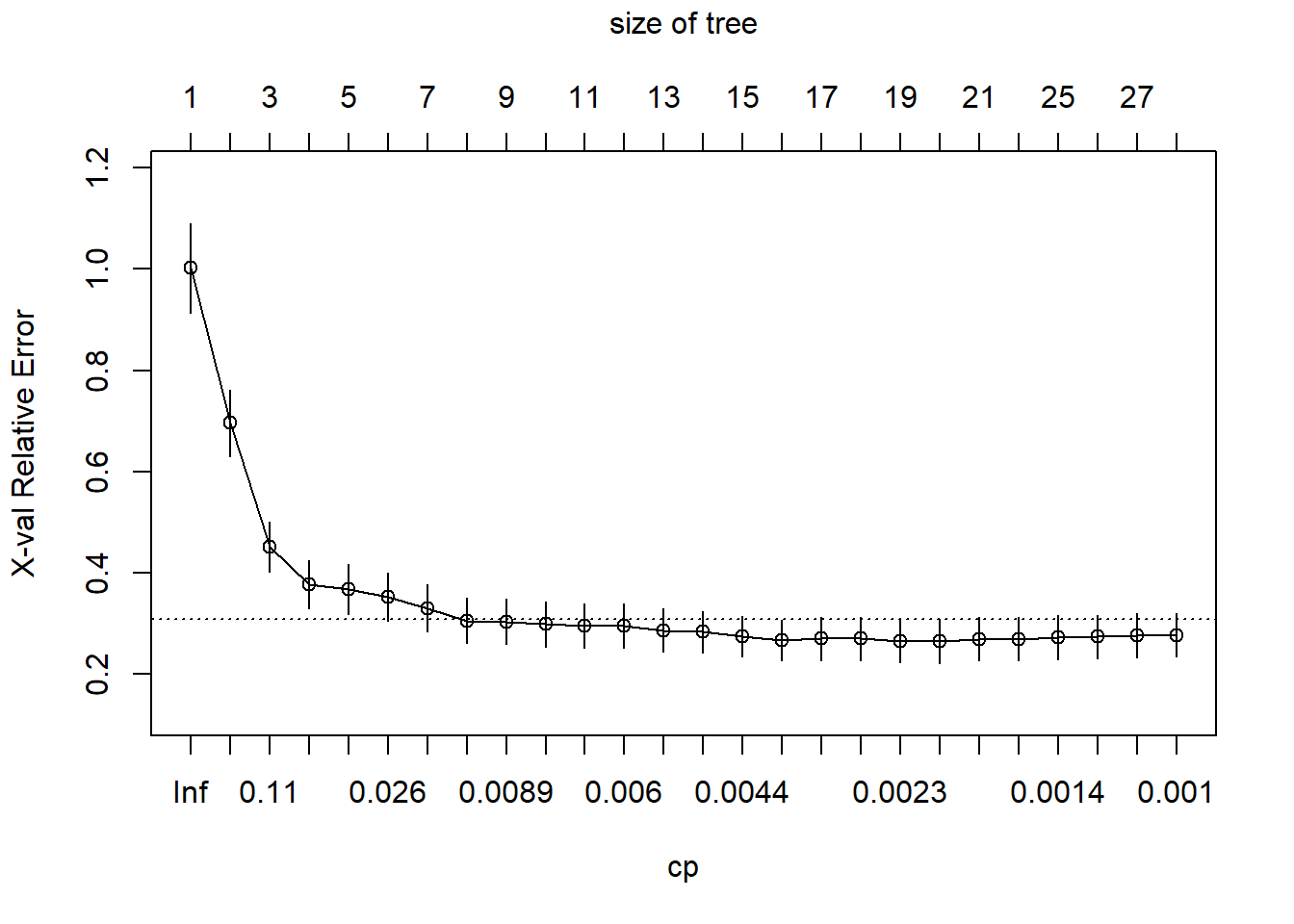In this lab we will go through the model building, validation, and interpretation of tree models. The focus will be on rpart package.

# 1 CART: classification and regression trees

CART stands for classification and regression trees:

• Regression trees: response variable Y is numerical
• Classification trees: response variable Y is categorical

For the regression trees example, we will use the Boston Housing data. Recall the response variable is the housing price. For the classification trees example, we will use the credit scoring data. The response variable is whether the loan went to default.

Note that unlkie logistic regreesion, the response variable does not have to be binary in case of classification trees. We can use classification trees on classification problems with more than 2 outcomes.

go to top

## 1.1 Regression Trees (Boston Housing Data)

Let us load the data sets. Random sampled training and test datasets will lead to different results,

library(MASS) #this data is in MASS package
boston_data <- data(Boston)
sample_index <- sample(nrow(Boston),nrow(Boston)*0.90)
boston_train <- Boston[sample_index,]
boston_test <- Boston[-sample_index,]

We will use the ‘rpart’ library for model building and ‘rpart.plot’ for plotting.

install.packages('rpart')
install.packages('rpart.plot') 
library(rpart)
library(rpart.plot)

The simple form of the rpart function is similar to lm and glm. It takes a formula argument in which you specify the response and predictor variables, and a data argument in which you specify the data frame.

boston_rpart <- rpart(formula = medv ~ ., data = boston_train)

### 1.1.1 Printing and ploting the tree

boston_rpart
## n= 455
##
## node), split, n, deviance, yval
##       * denotes terminal node
##
##  1) root 455 38119.4600 22.52440
##    2) lstat>=9.63 268  6453.8600 17.46828
##      4) lstat>=15 145  2834.0930 14.71793
##        8) crim>=5.76921 69   981.6325 11.94928 *
##        9) crim< 5.76921 76   843.3442 17.23158 *
##      5) lstat< 15 123  1229.8960 20.71057 *
##    3) lstat< 9.63 187 14995.5100 29.77059
##      6) rm< 7.437 161  6854.3400 27.27764
##       12) rm< 6.6565 100  3123.5690 24.49700
##         24) dis>=2.04295 93  1085.7160 23.63011 *
##         25) dis< 2.04295 7  1039.4290 36.01429 *
##       13) rm>=6.6565 61  1690.0410 31.83607
##         26) lstat>=5.495 28   425.9000 29.00000 *
##         27) lstat< 5.495 33   847.8406 34.24242 *
##      7) rm>=7.437 26   944.6785 45.20769 *
prp(boston_rpart,digits = 4, extra = 1)Make sure you know how to interpret this tree model!

Exercise: What is the predicted median housing price (in thousand) given following information:

crim zn indus chas nox rm age dis rad tax ptratio black lstat medv
0.08 0 12.83 0 0.44 6.27 6 4.25 5 398 18.7 394.92 6.78 24.1

### 1.1.2 Prediction using regression trees

The in-sample and out-of-sample prediction for regression trees is also similar to lm and glm models.

• In-sample prediction
boston_train_pred_tree = predict(boston_rpart)
• Out-of-sample prediction
boston_test_pred_tree = predict(boston_rpart,boston_test)

We often denote MSE as training error, and MSPE as testing error when sample size is large.

Exercise: Calculate the mean squared error (MSE) for this tree model

MSE.tree<-
MSPE.tree <- 

We can compare this model’s out-of-sample performance with the linear regression model with all variables in it.

boston.reg = lm(medv~., data = boston_train)
boston_test_pred_reg = predict(boston.reg, boston_test)
mean((boston_test_pred_reg - boston_test$medv)^2) ##  13.97123 ### 1.1.3 Comparing the performance of regression trees with linear regression model in terms of prediction error (Exercise) Calculate the mean squared error (MSE) and mean squared prediction error (MSPE) for linear regression model using all variables. Then compare the results. What is your conclusion? Further, try to compare the regression trees with the best linear regression model using some variable selection procedures. boston_lm<- boston_train_pred_lm<- boston_test_pred_lm<- MSE_lm<- MSPE_lm<- ## 1.2 Pruning In rpart(), the cp(complexity parameter) argument is one of the parameters that are used to control the compexity of the tree. The help document for rpart tells you “Any split that does not decrease the overall lack of fit by a factor of cp is not attempted”. For a regression tree, the overall R-square must increase by cp at each step. Basically, the smaller the cp value, the larger (complex) tree rpart will attempt to fit. The default value for cp is 0.01. What happens when you have a large tree? The following tree has 27 splits. boston_largetree <- rpart(formula = medv ~ ., data = boston_train, cp = 0.001) Try plot it yourself to see its structure. prp(boston_largetree) The plotcp() function gives the relationship between 10-fold cross-validation error in the training set and size of tree. plotcp(boston_largetree)You can observe from the above graph that the cross-validation error (x-val) does not always go down when the tree becomes more complex. The analogy is when you add more variables in a regression model, its ability to predict future observations not necessarily increases. A good choice of cp for pruning is often the leftmost value for which the mean lies below the horizontal line. In the Boston housing example, you may conclude that having a tree mode with more than 10 splits is not helpful. To look at the error vs size of tree more carefully, you can look at the following table: printcp(boston_largetree) ## ## Regression tree: ## rpart(formula = medv ~ ., data = boston_train, cp = 0.001) ## ## Variables actually used in tree construction: ##  age crim dis indus lstat nox ptratio rm tax ## ## Root node error: 38119/455 = 83.779 ## ## n= 455 ## ## CP nsplit rel error xerror xstd ## 1 0.4373118 0 1.00000 1.00164 0.088300 ## 2 0.1887878 1 0.56269 0.69598 0.065468 ## 3 0.0626942 2 0.37390 0.45100 0.049788 ## 4 0.0535351 3 0.31121 0.37745 0.047010 ## 5 0.0264725 4 0.25767 0.36746 0.050010 ## 6 0.0261920 5 0.23120 0.35175 0.047637 ## 7 0.0109209 6 0.20501 0.33029 0.047045 ## 8 0.0090019 7 0.19409 0.30502 0.044677 ## 9 0.0087879 8 0.18508 0.30392 0.044680 ## 10 0.0071300 9 0.17630 0.29857 0.044509 ## 11 0.0062146 10 0.16917 0.29601 0.043337 ## 12 0.0057058 11 0.16295 0.29607 0.043394 ## 13 0.0052882 12 0.15725 0.28684 0.042187 ## 14 0.0050891 13 0.15196 0.28323 0.040676 ## 15 0.0038747 14 0.14687 0.27419 0.040449 ## 16 0.0027861 15 0.14299 0.26735 0.039530 ## 17 0.0027087 16 0.14021 0.27024 0.042992 ## 18 0.0024745 17 0.13750 0.27029 0.042991 ## 19 0.0021641 18 0.13502 0.26574 0.042955 ## 20 0.0017623 19 0.13286 0.26513 0.042962 ## 21 0.0014982 20 0.13110 0.26924 0.042993 ## 22 0.0014823 22 0.12810 0.26936 0.042996 ## 23 0.0013043 24 0.12514 0.27291 0.043153 ## 24 0.0012656 25 0.12383 0.27368 0.043128 ## 25 0.0010158 26 0.12257 0.27658 0.043128 ## 26 0.0010000 27 0.12155 0.27720 0.043132 Root node error is the error when you do not do anything too smart in prediction, in regression case, it is the mean squared error(MSE) if you use the average of medv as the prediction. Note it is the same as sum((boston_train$medv - mean(boston_train$medv))^2)/nrow(boston_train) ##  83.77903 The first 2 columns CP and nsplit tells you how large the tree is. rel.error $$\times$$ root node error gives you the in sample error. For example, The last row (rel error)*(root node error)= 0.13085*87.133 = 11.40135, which is the same as the in-sample MSE if you calculate using predict: mean((predict(boston_largetree) - boston_train$medv)^2)
##  10.18341

xerror gives you the cross-validation (default is 10-fold) error. You can see that the rel error (in-sample error) is always decreasing as model is more complex, while the cross-validation error (measure of performance on future observations) is not. That is why we prune the tree to avoid overfitting the training data.

The way rpart() does it is that it uses some default control parameters to avoid fitting a large tree. The main reason for this approach is to save computation time. For example by default rpart set a cp = 0.1 and the minimum number of observations that must exist in a node to be 20. Use ?rpart.control to view these parameters. Sometimes we wish to change these parameters to see how more complex trees will perform, as we did above. If we have a larger than necessary tree, we can use prune() function and specify a new cp:

prune(boston_largetree, cp = 0.008)
## n= 455
##
## node), split, n, deviance, yval
##       * denotes terminal node
##
##  1) root 455 38119.4600 22.52440
##    2) lstat>=9.63 268  6453.8600 17.46828
##      4) lstat>=15 145  2834.0930 14.71793
##        8) crim>=5.76921 69   981.6325 11.94928 *
##        9) crim< 5.76921 76   843.3442 17.23158 *
##      5) lstat< 15 123  1229.8960 20.71057 *
##    3) lstat< 9.63 187 14995.5100 29.77059
##      6) rm< 7.437 161  6854.3400 27.27764
##       12) rm< 6.6565 100  3123.5690 24.49700
##         24) dis>=2.04295 93  1085.7160 23.63011
##           48) rm< 6.124 25   129.7400 20.50000 *
##           49) rm>=6.124 68   620.9851 24.78088 *
##         25) dis< 2.04295 7  1039.4290 36.01429 *
##       13) rm>=6.6565 61  1690.0410 31.83607
##         26) lstat>=5.495 28   425.9000 29.00000 *
##         27) lstat< 5.495 33   847.8406 34.24242
##           54) dis>=4.184 26   199.3154 32.56923 *
##           55) dis< 4.184 7   305.3771 40.45714 *
##      7) rm>=7.437 26   944.6785 45.20769 *

Exercise: Prune a classification tree. Start with “cp=0.001”, and find a reasonable cp value, then obtain the pruned tree.

Some software/packages can automatically prune the tree.

go to top